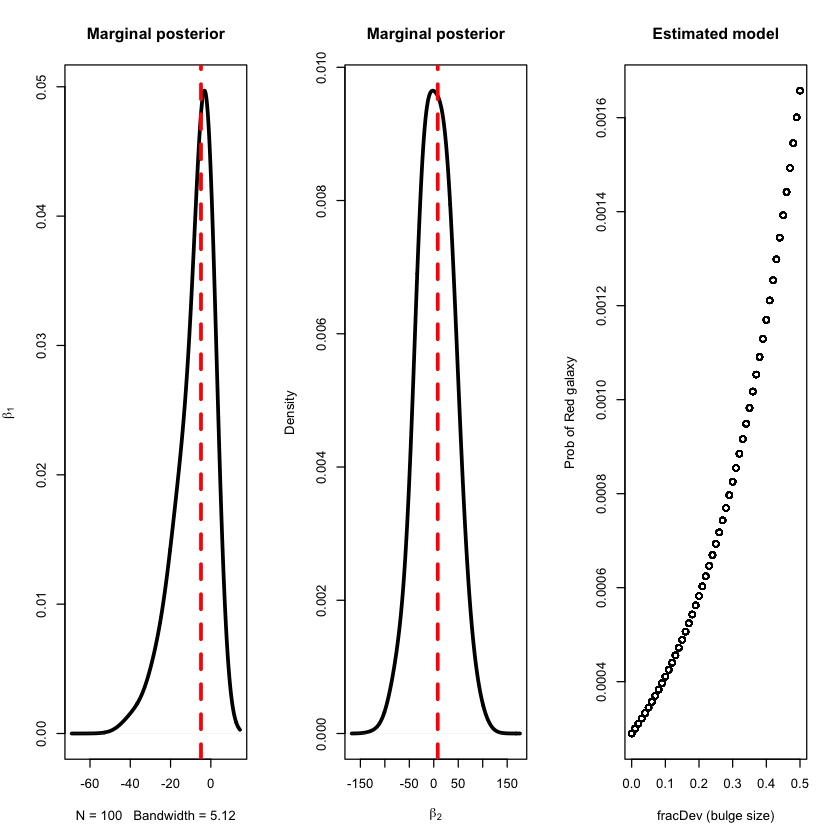# Approximate Bayesian Computation (ABC) Lab¶

## Problem 1: Binomial example¶

The data are a sample of 1's and 0's coming from $Y_i \sim \text{Bernoulli}(p)$ where $n$ = sample size, $\theta = P(Y = 1)$. If $Y = \sum_{i = 1}^n Y_i$, the $Y \sim \text{Binomial}(n,\theta)$ with a likelihood defined as

$$p(\theta \mid y) = {n \choose y}\theta^y(1 - \theta)^{n-y},$$

where $y = \sum_{i = 1}^ny_i$. We are going to pretend we do not know the likelihood function, and consider the following Bayesian model for this problem:

\begin{align*} Y &\sim \text{Binomial}(n,\theta) \\ \nonumber \theta &\sim \text{Uniform}(0,1) \end{align*}

## R Code¶

These are the parameters you'll need to in order to initialize the ABC but then you'll change them one at at time in the exercises below the code.

In :
set.seed(123)
n <- 20  #number of observations
N <- 100 #generated sample size
true.theta <- .75 #true parameter value
data <- rbinom(n,1,true.theta)
epsilon=0 	#Tolerance


The distance function, hyper parameters, and variables

In :
rho <- function(y,x) abs(sum(y)-sum(x))/n
alpha.hyper <- 1 #Hyper parameter
beta.hyper <- 1 #Hyper parameter
theta <- numeric(N) #"Good" proposals stored in theta


The ABC algorithm. Note that this is not setup to be computationally efficient, but rather to be easier to understand what is going on in the code.

In :
for(i in 1:N){
d=epsilon+1
while(d>epsilon) {
proposed.theta <- rbeta(1,alpha.hyper,beta.hyper)
x <- rbinom(n,1,proposed.theta)
d <- rho(data,x)
}
theta[i] <-  proposed.theta
}


Plots of the ABC posterior and true posterior

In :
grid0 <- seq(0, 1, length.out = 1000)
par(mar <- c(4,4,2,2), oma = c(2,2,2,2))
hist(theta, main = paste("Tolerance: ", epsilon, ", N:", N, ", n:",n, sep = ""), nclass = 25, freq = FALSE, xlim = c(0,1))
den1 <- density(theta)
lines(den1$x,den1$y,col = "black",lwd = 4)
abline(v = true.theta, col = "red", lty = 2, lwd = 4)
lines(grid0,dbeta(grid0,sum(data)+1,n-sum(data)+1),col = "blue",lwd = 4)
legend("topleft", c("True Posterior","ABC Posterior",paste("True theta: ", true.theta, sep = "")), col = c("blue","black","red"), lty = c(1,1,2),lwd = 3)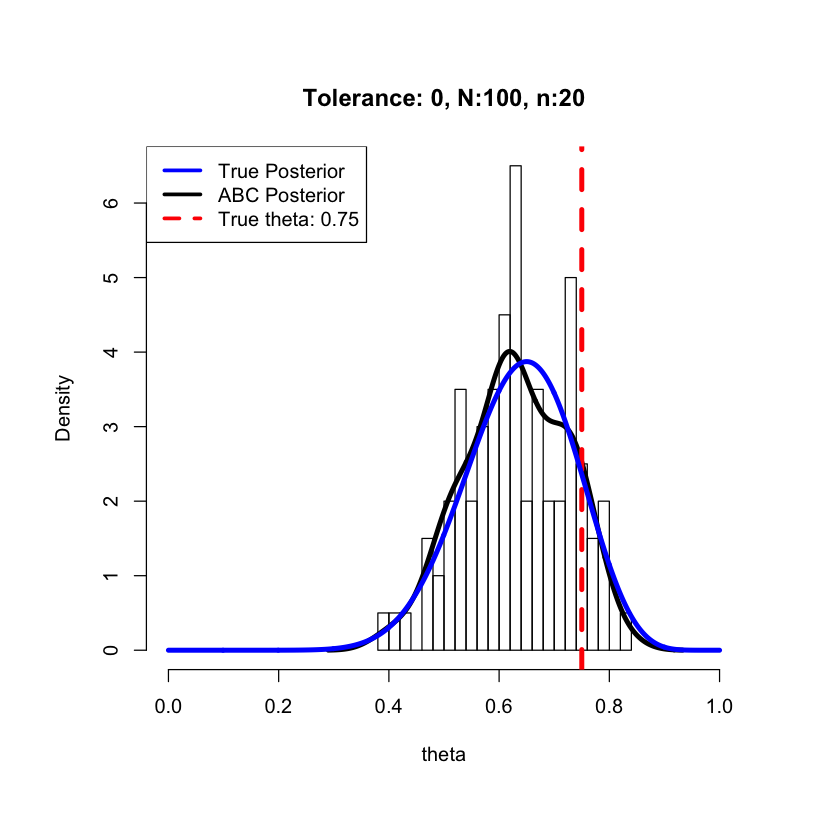### Exercises¶

1. The sample size, $\texttt{n}$, is currently set to 20. Try running the code with different values for $\texttt{n}$ (e.g. $\texttt{n = 10, 50, 100}$). What happens to the true posterior as $\texttt{n}$ increases? What happens as $\texttt{n}$ decreases?

2. Set $\texttt{n=20}$. The particle sample size, $\texttt{N}$, is currently set to 100. Try running the code with different values for $\texttt{N}$ (e.g. $\texttt{N = 25, 200, 500}$). What happens to the ABC posterior as $\texttt{N}$ increases? What happens as $\texttt{N}$ decreases?

3. Set $\texttt{N = 200}$. The tolerance, $\texttt{epsilon}$, is currently set to 0. Try running the code with different values for $\texttt{epsilon}$ (e.g. $\texttt{epsilon = 0.01, 0.1, 1}$). What conclusions can you draw?

4. The probability, $\texttt{theta}$, is currently set to 0.75. Try running the code with different values for $\texttt{theta}$ (between 0 and 1 since it is a probability). What happens to the true posterior as you change this?

5. The distance function, $\texttt{rho}$, is currently set to be $\rho(y,x) = |\sum_{i = 1}^n y_i - \sum_{i = 1}^nx_i|/n$. Try running the code without the absolute value in the distance function (i.e. $\rho(y,x) = (\sum_{i = 1}^n y_i - \sum_{i = 1}^nx_i)/n$). What happens to the ABC-posterior? Why does this happen? Hint: consider the values of the means of the generated data (i.e., the generated $\hat{theta} = n^{-1}\sum_{i = 1}^n x_i$) that are going to be accepted with this distance function.

## Problem 2: Gaussian example¶

Suppose we have a sample $i = 1, \ldots, n$ of $Y_i \sim N(\mu, \sigma^2)$ where $\mu$ is unknown and $\sigma^2$ is known. Consider the following Bayesian model:

\begin{align*} \mu &\sim N(\mu_0, \sigma^2_0) \nonumber \\ Y_i\mid \mu, \sigma^2 &\sim N(\mu, \sigma^2). \end{align*}

Then the posterior for $\mu$ is $\pi(\mu \mid y_{1:n}) \sim N(\mu_1, \sigma_1^2)$ where

$$\mu_1 = \frac{\left(\frac{\mu_0}{\sigma^2_0} + \frac{\sum y_i}{\sigma^2}\right)}{\left(\frac{1}{\sigma_0^2} + \frac{n}{\sigma^2}\right)},\qquad \sigma_1^2 = \frac{1}{\left(\frac{1}{\sigma_0^2} + \frac{n}{\sigma^2}\right)}$$

## R Code¶

These are the parameters you'll need to in order to initialize the ABC but then you'll change them one at at time in the exercises below the code.

In :
set.seed(123)
n <- 25 #number of observations
N <- 100 #particle sample size
true.mu <- 0 #true parameter value (considered unknown)
sigma <- 1 #true parameter value (considered known)


The distance function, hyper parameters, and variables

WARNING: when you change the distance function, it is a good idea to start by running it with a larger epsilon

In :
rho <- function(y,x) abs(sum(y)-sum(x))/n
epsilon <- 0.05 #Tolerance
mu.hyper <- 0 #Hyper parameter
sigma.hyper <- 10
data <- rnorm(n,true.mu,sigma)
mu <- numeric(N) #"Good" proposals stored in mu


The ABC algorithm. Note that this is not setup to be computationally efficient, but rather to be easier to understand what is going on in the code.

In :
for(i in 1:N){
d <- epsilon +1
while(d>epsilon) {
proposed.mu <- rnorm(1,0,sigma.hyper) # prior draw
x <- rnorm(n, proposed.mu, sigma)
d <- rho(data,x)
}
mu[i] <- proposed.mu
}


Plots of the ABC posterior and true posterior

In :
grid0 <- seq(-1, 1, length.out = 1000)
mu1 <- (mu.hyper/sigma.hyper^2 + sum(data)/sigma^2)/(1/sigma.hyper^2 + n/sigma^2)
sigma1 <- sqrt(1/(1/sigma.hyper^2 + n/sigma^2))
par(mar = c(4,4,2,2), oma = c(2,2,2,2))
hist(mu, main = paste("Tolerance: ", epsilon, ", N:", N, ", n:",n, sep = ""), nclass = 25, freq = FALSE, xlim = c(-1,1))
den1 <- density(mu)
lines(den1$x,den1$y,col = "black",lwd = 4)
abline(v = true.mu, col = "red", lty = 2, lwd = 4)
lines(grid0,dnorm(grid0,mu1,sigma1),col = "blue",lwd = 4)
legend("topright", c("True Posterior","ABC Posterior","True mu"), col = c("blue","black","red"), lty = c(1,1,2),lwd = 3)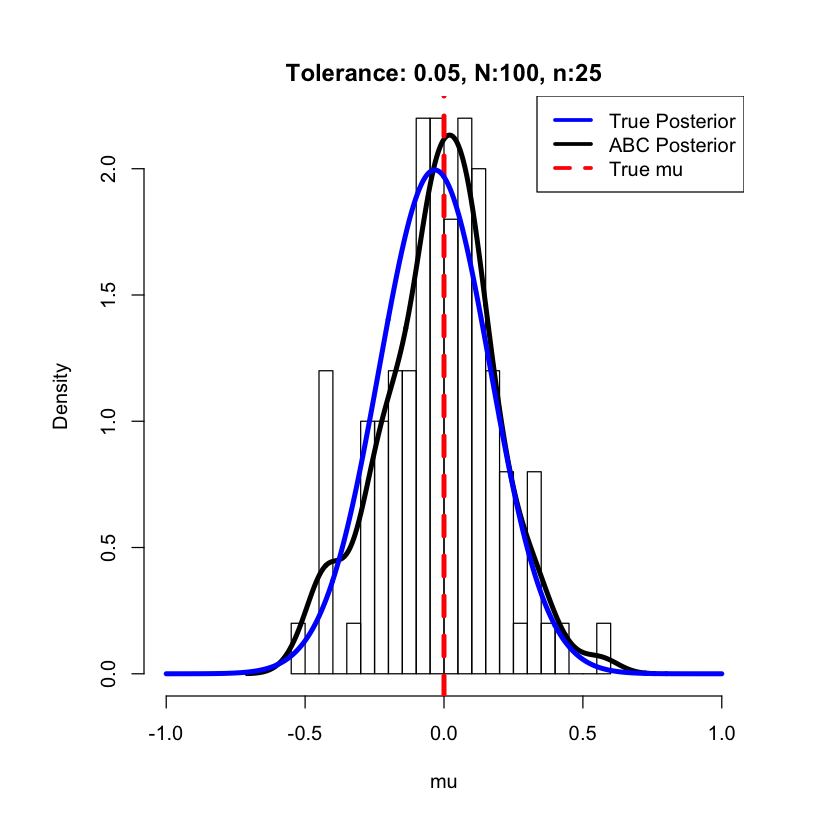### Exercises¶

1. The tolerance, $\texttt{epsilon}$, is currently set to 0.05. Try running the code with different values for $\texttt{epsilon}$. What conclusions can you draw?

2. Set $\texttt{epsilon = 0.005}$. The true value for $\mu$ is currently set at 0 and $\sigma^2$ is 1. What happens to the true posterior as you change these values?

3. The distance function, $\texttt{rho}$, is currently set to be $\rho(y,x) = |\sum_{i = 1}^n y_i - \sum_{i = 1}^nx_i|/n$. Try different distance functions to see what happens (e.g. $\rho(y,x) = (\sum_{i = 1}^n y_i - \sum_{i = 1}^nx_i)^2/n$, KS distance with $\texttt{R}$ function $\texttt{ks.test}$). What happens to the ABC-posterior? Why does this happen? How does it impact the tolerances?

4. Remove the assumption that $\sigma^2$ is known. This means you will have to (1) Determine an appropriate prior distribution on $\sigma^2$ (e.g. inverse-gamma, uniform), (2) Pick an appropriate summary statistic (hint: find a sufficient statistic for $\sigma^2$), (3) Set-up a 2-dimensional distance function and a 2-dimensional tolerance to account for the two summary statistics.

## Problem 3: Sequential Gaussian Example¶

Using the same model as Problem 2, suppose we have a sample $i = 1, \ldots, n$ of $Y_i \sim N(\mu, \sigma^2)$ where $\mu$ is unknown and $\sigma^2$ is known. Consider the following Bayesian model:

\begin{align*} \mu &\sim N(\mu_0, \sigma^2_0) \nonumber \\ Y_i\mid \mu, \sigma^2 &\sim N(\mu, \sigma^2). \end{align*}

## R Code¶

These are the parameters you'll need to in order to initialize the ABC, including the distance function, hyper parameters, and variables.

In :
set.seed(123)
time.steps <- 10 #Here you can change the number of time steps in the sequential algorithm.
rho <- function(y,x) abs(sum(y)-sum(x))/n
n <- 25 # number of observations
N <- 100 # particle sample size
true.mu <- 0 # true parameter value (considered unknown)
sigma <- 1 # true parameter value (considered known)
mu.hyper <- 0 # Hyper parameter
sigma.hyper <- 10 # Hyper parameter
data <- rnorm(n,true.mu,sigma)
epsilon <- 1 # Starting tolerance

weights <- matrix(1/N,time.steps,N) # Importance weights
mu <- matrix(NA,time.steps,N)
d <- matrix(NA,time.steps,N)


The ABC-PMC algorithm. Note that this is not setup to be computationally efficient, but rather to be easier to understand what is going on in the code.

In :
for(t in 1:time.steps){
print(t)
if(t==1){
for(i in 1:N){
d[t,i] <- epsilon +1
while(d[t,i]>epsilon) {
proposed.mu <- rnorm(1,0,sigma.hyper) #<--prior draw
x <- rnorm(n, proposed.mu, sigma)
d[t,i] <- rho(data,x)
}
mu[t,i] <- proposed.mu
}} else{
epsilon <- c(epsilon,quantile(d[t-1,],.75))
mean.prev <- sum(mu[t-1,]*weights[t-1,])
var.prev <- sum((mu[t-1,] - mean.prev)^2*weights[t-1,])
for(i in 1:N){
d[t,i] <- epsilon[t]+1
while(d[t,i]>epsilon[t]) {
sample.particle <- sample(N, 1, prob = weights[t-1,])
proposed.mu0 <- mu[t-1, sample.particle]
proposed.mu <- rnorm(1, proposed.mu0, sqrt(2*var.prev))
x <- matrix(rnorm(n,proposed.mu, sigma),n,1)
d[t,i]=rho(data,x)
}
mu[t,i]= proposed.mu
mu.weights.denominator <- sum(weights[t-1,]*dnorm(proposed.mu, mu[t-1,],sqrt(2*var.prev)))
mu.weights.numerator <- dnorm(proposed.mu,0,sigma.hyper)
weights[t,i] <- mu.weights.numerator/mu.weights.denominator
}}
weights[t,] <- weights[t,]/sum(weights[t,])
}

 1
 2
 3
 4
 5
 6
 7
 8
 9
 10


Plots of the ABC posterior and true posterior

In :
library(fields) # Install the library fields,' and then load it here (for the tim.colors)
col1 <- tim.colors(time.steps)

grid0 <- seq(-1, 1, length.out = 1000)
mu1 <- (mu.hyper/sigma.hyper^2 + sum(data)/sigma^2)/(1/sigma.hyper^2 + n/sigma^2)
sigma1 <- sqrt(1/(1/sigma.hyper^2 + n/sigma^2))

time.step <-1   # Change the time step here

den1 <- density(mu[time.step,],weights = weights[time.step,])
plot(den1$x,den1$y,"l", col = col1[time.step],lwd = 3, xlab = "mu", ylab = "Density", ylim = c(0,2.5))
abline(v = true.mu, col = "red", lty = 2, lwd = 3)
den1 <- density(mu[time.step,],weights = weights[time.step,])
lines(den1$x,den1$y,"l", col = "magenta",lwd = 8)
lines(grid0,dnorm(grid0,mu1,sigma1),col = "black",lwd = 5)
legend("topright", c("True Posterior","ABC Posterior", "True mu"), col = c("black","magenta","red"), lty = c(1,1,2),lwd = 3)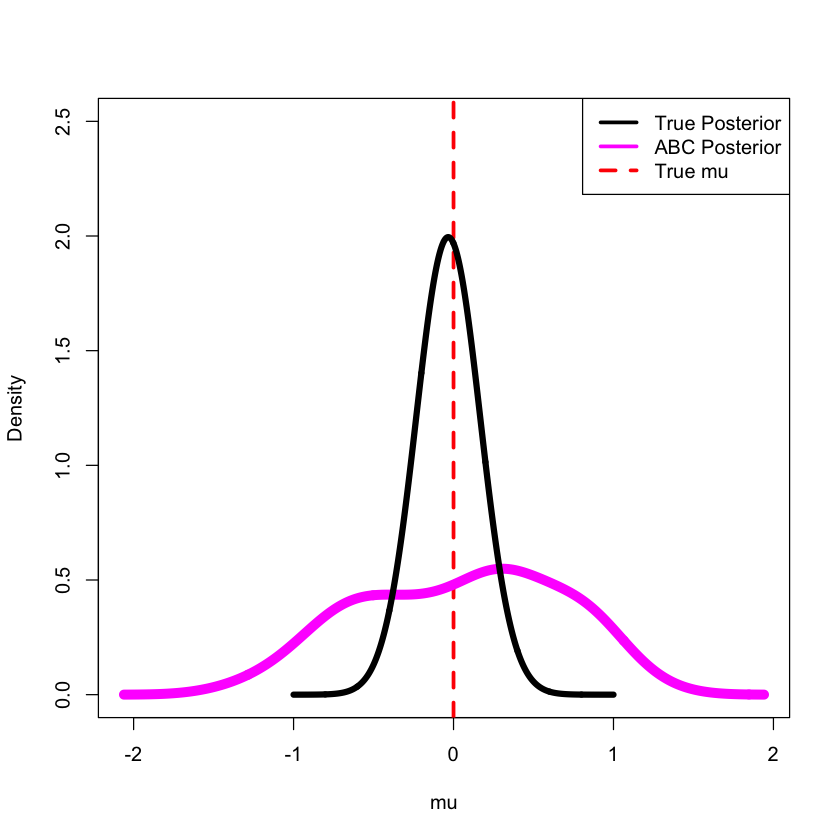Plots of all the sequential ABC posterior and true posterior

In :
library(fields) # Install the library fields,' and then load it here (for the tim.colors)
col1 <- tim.colors(time.steps)

grid0 <- seq(-1, 1, length.out = 1000)
mu1 <- (mu.hyper/sigma.hyper^2 + sum(data)/sigma^2)/(1/sigma.hyper^2 + n/sigma^2)
sigma1 <- sqrt(1/(1/sigma.hyper^2 + n/sigma^2))

par(mfrow = c(1,1), mar = c(4,4,2,2), oma = c(2,2,2,2))
den1 <- density(mu[1,],weights = weights[1,])
plot(den1$x,den1$y,"l", col = col1,lwd = 3, xlab = "mu", ylab = "Density", ylim = c(0,2.25))
for(which.t in 2:time.steps){
den1 <- density(mu[which.t,],weights = weights[which.t,])
lines(den1$x,den1$y,col = col1[which.t],lwd = 3)
}
abline(v = true.mu, col = "red", lty = 2, lwd = 3)
den1 <- density(mu[time.steps,],weights = weights[time.steps,])
lines(den1$x,den1$y,"l", col = "magenta",lwd = 8)
lines(grid0,dnorm(grid0,mu1,sigma1),col = "black",lwd = 5)
legend("topright", c("True Posterior","ABC Posterior", "True mu"), col = c("black","magenta","red"), lty = c(1,1,2),lwd = 3)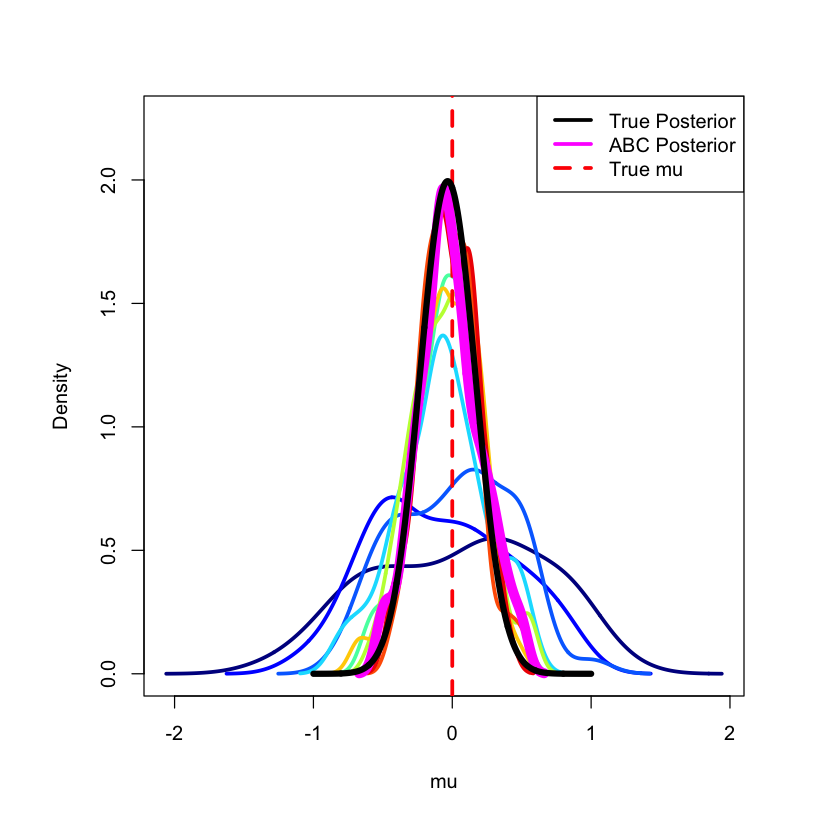Plots the decreasing tolerances

In :
plot(epsilon, pch = 19, lwd = 3, xlab = "Time step", ylab = "Tolerance")### Exercises¶

1. Run the code and plot the ABC - posteriors at different time steps. How do they compare?

2. There are currently 10 time steps in the algorithm. Try changing this to something larger, say 25. Is there a significant improvement in the posterior at $t = 10$ versus $t = 20$? How are you deciding?

3. As in Problem (2), remove the assumption that $\sigma^2$ is known. Use the same set-up as before, but now you have to figure out how to do it sequentially. Use the updating kernel for $\mu$ as an example for how to update $\sigma^2$.

## Problem 4: Astronomical example (fraction of Red spirals)¶

This example was developed based on Section 10.6 of Hilbe, J. M., R. S. de Souza, and E. E. O. Ishida. "Bayesian Models for Astrophysical Data Using R." JAGS, Python, and Stan (2017) where they develop a model for the probability of a galaxy being a red spiral galaxy as a funciton of the size of the galaxy bulge.

Using the notaion of Hilbe et al (2017), they define the model as

\begin{align*} & T_i \sim \text{Bernoulli}(p_i) \\ \nonumber & \log\left(\frac{p_i}{1-p_i}\right) = \eta_i \\ \nonumber & \eta_i = \beta_1 + \beta_2 x_i \\ \nonumber & \beta_j \sim \text{Normal}(0, 10^3) \\ \nonumber & i = 1, \ldots, n \\ \nonumber & j = 1, \ldots, K \\ \nonumber \end{align*}

They use a dataset that includes $n = 5433$ observations and $K = 2$. Notice that the linear predictor, $\eta_i$, can be expanded to include more predictors. The real observations are available here in the textbook's GitHub repository: https://github.com/astrobayes/BMAD/blob/master/data/Section_10p6/Red_spirals.csv The $x_i$ variable is the proxy for the bulge size which is the fraction of the best-fit light profile from the de Vaucouleurs fit'' and defined as $\texttt{fracdeV}$. The response is binary (1 or 0) where 1 indicates a red, passive spiral and a 0 indicates a blue, active, spiral.

## R Code¶

We will need the library(mvtnorm) in order to compute the importance weights for the parameters.

In :
install.packages("mvtnorm", repos='http://cran.us.r-project.org')
require(mvtnorm)

The downloaded binary packages are in

In :
set.seed(87654)
library(mvtnorm)


The algorithm parameters are set here. The $\texttt{Ndraws}$ (the number of particles) and $\texttt{T_Iter}$ (the number of iterations) both can/should be set to a much larger value.

In :
Ndraws <- 100 # number of particles
Ninit <- Ndraws*5  # number of initial particles
T_Iter <- 3  # number of iterations
which_quantile <- .25  # quantile for decreasing tolerance

theta_prior <- cbind(c(0,0), c(10^3, 10^3))
colnames(theta_prior) <- c("Mean", "Var")


The forward model is defined based on the Bayesian model described above. The inputs are $\texttt{theta}$ (in this case, $\beta_1$ and $\beta_2$) and $\texttt{x}$ (the $\texttt{Fracdev}$).

In :
forwardModel <- function(theta,x){
eta <- theta + theta*x
y_prob <- 1/(1+exp(-eta))
y_proposed <- rbinom(length(x),1,y_prob)
return(y_proposed)
}


The distance function is currently set to compare the sample means of the real and proposed observations. You may want to consider changing this to see what happens.

In :
getDistance <- function(y1,y2){
distance_xy <- abs(mean(y1) - mean(y2))
return(distance_xy)
}


Read in the observations - you will need to be sure the dataset is in your working directory. The two variables we are interested in are the galaxy type (1 = red, passive, spirals, 0 = blue, active, spirals), and the proxy for the bulge size (the fraction of the best-fit light profile from the de Vaucouleurs fit'' and defined as $\texttt{fracdeV}$).

In :
Red <- read.csv("Red_spirals.csv", header = TRUE)
dim(Red)
x <- Red$fracdeV y <- Red$type

theta_true <- c(-4.89, 8.11) # These aren't necessarily the true values,
# but the estimates from the MCMC approach in Hilbe et al (2017)
# y <- forwardModel(theta_true, x)

redshiftg.rg.r_errfracdeVtype
0.08330.695 0.020 0.42 1
0.07350.701 0.021 0.46 1
0.06420.756 0.039 0.00 1
0.06210.662 0.017 0.32 1
0.06610.687 0.018 0.40 1
0.05840.691 0.026 0.00 1
1. 5433
2. 5

In this case, we initialize the algorithm adaptively. That is, rather than specifying a fixed tolerance, we are going to generate $\texttt{Ninit > Ndraws}$ values and then keep the $\texttt{Ndraws}$ with the smallest distance.

In :
# Output that we wish to store - could add more
post_theta <-NULL
post_weights <- NULL
post_dist <- NULL

t_Iter <- 1
theta_prop <- matrix(NA, ncol = 2, nrow = Ninit)
dist_y <- c()
for(ii in 1:Ninit){
#print(ii)
theta_prop[ii,1] <- rnorm(1,theta_prior[1,1], sqrt(theta_prior[1,2]))
theta_prop[ii,2] <- rnorm(1,theta_prior[2,1], sqrt(theta_prior[2,2]))
y_prop <- forwardModel(theta_prop[ii,], x)
dist_y[ii] <- getDistance(y, y_prop)
}


Since we are doing an adaptive start, we now need to find the particles that have the $\texttt{Ndraws}$ smallest distances. Then we save the various variables and determine the next tolerance.

In :
dist_rank <- rank(dist_y, ties.method = "random")
pickNdraws <- dist_rank <= Ndraws

Dvals2 <- dist_y[pickNdraws]

theta_current <- theta_prop[pickNdraws, ]

weights <- rep(1/Ndraws,Ndraws)
post_weights[[t_Iter]] <- weights
post_theta[[t_Iter]] <- matrix(theta_current, ncol = ncol(theta_current))

cat('Marginal posterior mean is', c(sum(post_theta[[t_Iter]][,1]*weights), sum(post_theta[[t_Iter]][,2]*weights)),'\n')

post_tol_seq <- max(Dvals2)
tol_seq <- quantile(Dvals2,which_quantile)
cat('New tolerance is', tol_seq,'\n')

Marginal posterior mean is -22.66083 -3.340864
New tolerance is 0.0481778


After completing the initial iteration, we are now able to proceed sequentially. The particles are drawn from the previous iteration's particle system and then moved according to a Gaussian kernel. More details about this sequential algorithm can be found in Beaumont, Cornuet, Marin, & Robert (2009). Adaptive approximate Bayesian computation. Biometrika, 96(4), 983-990. The importance weights are crucial and if you change how the particles are jittered, you also need to update the importance weight calculation.

In :
for(t_Iter in 2:T_Iter){
print(t_Iter)

theta_prop <- matrix(NA, ncol = 2, nrow = Ndraws)
dist_y <- c()
weights0 <- c()

prev_wmean <- t(theta_current)%*%weights
prev_center <- theta_current - matrix(rep(prev_wmean,Ndraws),byrow = T, nrow = Ndraws)
var_prev <- t(prev_center)%*%diag(weights)%*%prev_center

for(ii in 1:Ndraws){
dist0 <- tol_seq+1
while(dist0 > tol_seq){
index_samp <- sample(1:Ndraws, 1, prob = weights)
theta_gen0 <- theta_current[index_samp,]
theta_gen <- rmvnorm(1, theta_gen0, 2*var_prev)

y_prop <- forwardModel(theta_gen, x)
getDistance(y, y_prop)
dist0 <- getDistance(y, y_prop)
}

dist_y[ii] <- dist0

weights_den <- sum(weights*apply(matrix(theta_current,nrow = Ndraws), 1, dmvnorm, x = theta_gen, sigma = 2*var_prev, log=FALSE))
weights_num <- dmvnorm(theta_gen, theta_prior[,1], diag(theta_prior[,2]))
weights0[ii] <- weights_num/weights_den
theta_prop[ii,] <- theta_gen
}

theta_current <- theta_prop
weights <- weights0/sum(weights0)
post_weights[[t_Iter]] <- weights
post_theta[[t_Iter]] <- theta_current

cat('Marginal posterior mean is', c(sum(post_theta[[t_Iter]][,1]*weights), sum(post_theta[[t_Iter]][,2]*weights)),'\n')

tol_seq <- quantile(dist_y,which_quantile)
post_tol_seq <- c(post_tol_seq, tol_seq)
cat('New tolerance is', tol_seq,'\n')
}

 2
Marginal posterior mean is -8.177648 2.031687
New tolerance is 0.01500092
 3
Marginal posterior mean is -8.146335 3.491138
New tolerance is 0.005613841


Next we plot the resulting marginal ABC posteriors and the model that is fit using the posterior means.

In :
which_step <- t_Iter
par(mfrow = c(1,3))
plot(density(post_theta[[which_step]][, 1],weights = post_weights[[which_step]]), lwd = 3, ylab = expression(beta), main = "Marginal posterior")
abline(v = theta_true, col = "red",lty = 2, lwd = 3)
#
plot(density(post_theta[[which_step]][, 2],weights = post_weights[[which_step]]), lwd = 3, xlab = expression(beta), main = "Marginal posterior")
abline(v = theta_true, col = "red",lty = 2, lwd = 3)
#
eta <-  sum(post_theta[[which_step]][,1]*weights) + sum(post_theta[[which_step]][,2]*weights)*x
y_prob <- 1/(1+exp(-eta))
plot(x, y_prob, "p", xlab = "fracDev (bulge size)", ylab = "Prob of Red galaxy", main = "Estimated model")